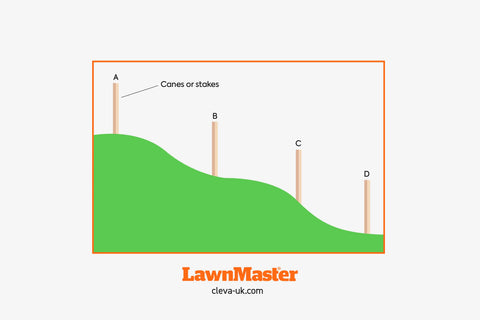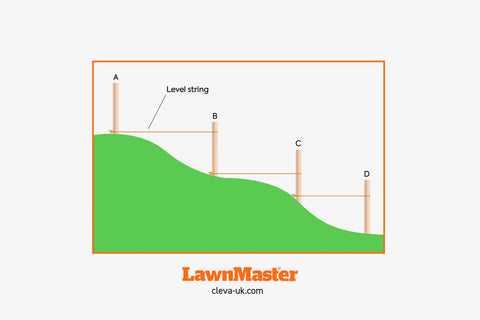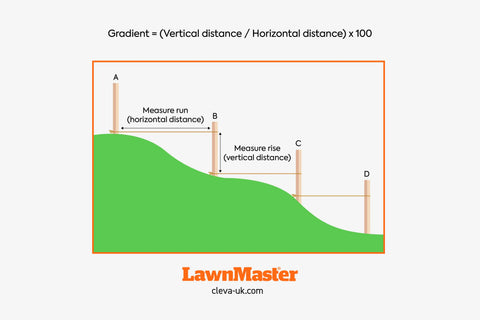02 Aug

# Robot mowers: How to measure the gradient or slope of your lawn

When choosing a robot lawn mower, it is important to understand the gradient or slope of your lawn. In this blog post, we will provide you with a step-by-step guide on how to accurately measure the gradient/slope of your garden as a percentage.

To measure the gradient, it is necessary to know the run or horizontal distance (level) along the slope, and the rise (the height of the slope).

### Step 1: Gather the necessary tools:

To measure the run and the rise, you will need the following tools:

Wooden stakes and string: These will be used to create reference points along the slope. Long bamboo canes are perfect for this task as they require very little effort to drive into the ground and are inexpensive for longer lengths.

Spirit level: A long spirit level, preferably at least 1m in length, to check the string between your reference canes is level.

Measuring tape: to measure the distance between your stakes/canes and the height of the rise.

### Step 2: Identify the starting and ending points:

Stake the ground at reference points that represent the area you wish to measure.

For a gentle slope over a smaller area, it may be possible to place stakes at the highest and lowest points, depending on the height of the stakes. However, be aware that the string line between the stakes may end up overly long and difficult to check whether it is level.

It may be more manageable to split the slope into 1m sections working from the highest point to the lowest, especially if the slope is uneven and steeper in some places than others. Place stakes or cane markers at these 1m reference points.### Step 3: Establish the reference line(s):

Tie a string tightly between the first two stakes, ensuring it is level. The string should run from the bottom of the upper cane (i.e. the start of the slope) to the cane below it (see diagram below), ensuring the string is level using the spirit level.

This string will act as your reference line for measuring the run (horizontal distance) and the rise (vertical distance) of the slope.

Repeat this process between any other canes that are placed along the slope.### Step 4: Measure the vertical distance (rise):

Measure from the string reference line on the stake or cane, to the ground. This is the rise.

### Step 5: Measure the horizontal distance (run):

If the canes have been placed 1m apart, it is worth taking a moment to double check this distance with the measuring tape. If the canes were placed at the top and bottom of the slope, measure this distance along the level string and note it down.### Step 6: Calculate the gradient:

To calculate the gradient, divide the vertical distance (rise) by the horizontal distance (run) and multiply by 100 to express it as a percentage. The formula is:

Gradient = (Vertical distance / Horizontal distance) x 100

For example, if the vertical distance is 30 cm and the horizontal distance is 150 cm, the gradient would be (30/150) x 100 = 20%.

If more than two reference points were used along the slope, the gradient can either be measured between each set of reference points, or alternatively all rise measurements can be added together and all run measurements can be added together, and the totals entered into the formula. This will give an average gradient for the overall slope. In cases where the slope is uneven and there are steeper sections this is a less reliable method.

Measuring the gradient/slope of your garden is crucial when choosing a robot lawnmower. For example, the LawnMaster L10 Robotic Mower navigates slopes up to 35% (19°) inside the boundary wire and slopes of up to 17% (10°) at the boundary wire.

By following the step-by-step guide outlined above, you can be sure to accurately determine the slope of your garden.

From our highly popular LawnMaster VBRM16 ‘Drop and Mow’ Robot Mower, to our larger L10 and L12 Robotic Lawnmowers, whatever the shape or size of your garden, we’ll help you to find the best robot mower for you. View the LawnMaster Robot Lawnmower range.

Posted by